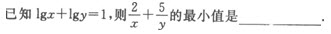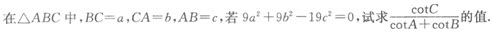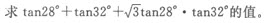# 2021年成人高考高起点《文数)》模拟试题四

2021-03-01 15:48 江西成考网

1.由0，1，2，3，4五个数字组成没有重复数字的五位偶数的个数为（   ）

A.120个
B.60个
C.36个
D.24个

2.A.48/5
B.183
C.12
D.10√7-14

3.（   ）

A.x2+y2-2x-2y-2=0
B.x2+y2-2x-2y+2=0
C.x2+y2+2x-2y-2=0
D.x2+y2-2x+2y+2=0

4.（   ）

A.x∈R
B.x∈R，且x≠2
C.x∈R，且x≠0
D.0<x<2

5.（    ）

A.4
B.√2
C.1/2
D.2

6.（   ）

A.10
B.±10
C.10或-68
D.23或-81

7.下列函数为偶函数的是（   ）

A.y=-x
B.y=x sinx
C.y=x cosx
D.y=x2+x

8.（   ）

A.370
B.270
C.250
D.490

9.（   ）

A.B./3
C.-D.-/3

10.（   ）

A.[0,12]
B.[0,11]
C.[-1,1]
D.[5,10]

11.直线2x+3y-6=0关于y轴对称的直线方程是（   ）

A.2x-3y-6=0
B.2x-3y+6=0
C.2x+3y+6=0
D.2x+3y-6=0

12.（   ）

A.-5/2
B.-7/2
C.-4
D.-2

13.由1，2，3，4组成的无重复数字的四位数，按从小到大的顺序排成一个数列{an)，则 a18等于（　　）

A.1243
B.3421
C.4123
D.3412

14.设函数y=ax+2的反函数为它的自身，则a的值是（   ）

A.±1
B.1
C.-1
D.4

15.A.{2,3}
B.{3,2}
C.{(2,5)}
D.{(5,2)}

16.下列各不等式中，同解的是（   ）

A.B.C.D.17.下列函数中，为偶函数且区间在（0，+∞）上单调递减的函数是（   ）

A.B.C.D.18.759
2022年成人高考考试时间
10月15,16日+